# D Question 19 1 pts When is a system whose transfer function is an improper rational...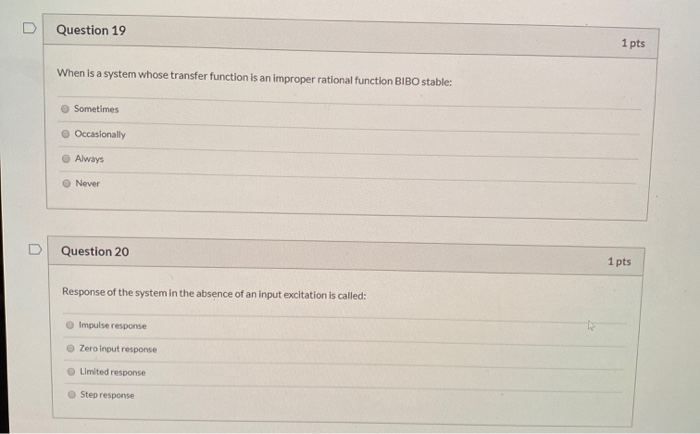D Question 19 1 pts When is a system whose transfer function is an improper rational function BIBO stable: Sometimes Occasionally Always Never Question 20 1 pts Response of the system in the absence of an input excitation is called: Impulse response Zero input response Limited response Step response

Ans 19) A system whose transfer function is improper rational( order of numerator > order of denominator) function is not BIBO(Vounded Input Bounded Output) stable ,regardless of location of it's poles and zeroes.

so correct answer is option D( Never).

Ans 20) zero input response is the response of the system in the absence of input excitation.

##### Add Answer of: D Question 19 1 pts When is a system whose transfer function is an improper rational...
Similar Homework Help Questions
• ### When is a system whose transfer function is an improper rational function BIBO stable: Occasionally Sometimes...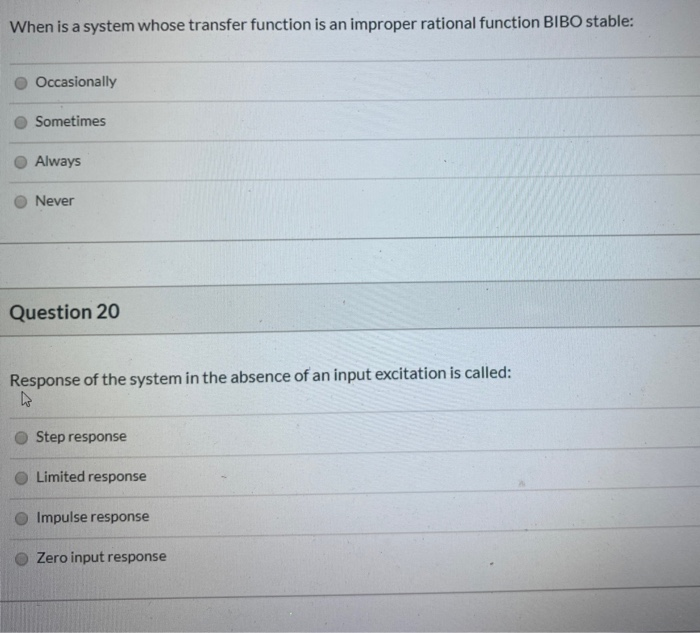When is a system whose transfer function is an improper rational function BIBO stable: Occasionally Sometimes Always Never Question 20 Response of the system in the absence of an input excitation is called: Step response Limited response Impulse response Zero input response

• ### Question 17 1 pts Knowing the transfer function of a system is useful because: O It...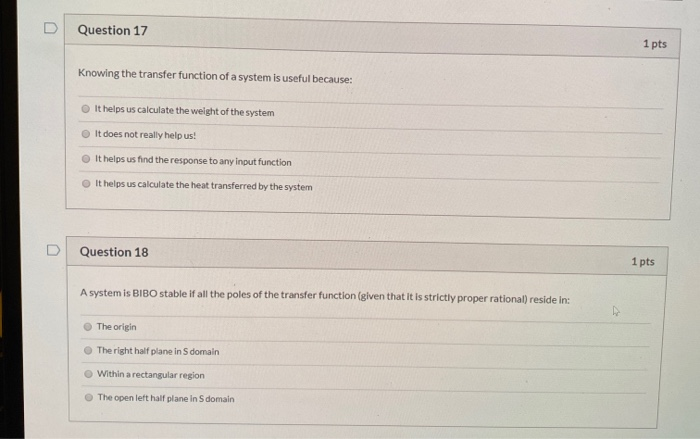Question 17 1 pts Knowing the transfer function of a system is useful because: O It helps us calculate the weight of the system It does not really help us! It helps us find the response to any input function It helps us calculate the heat transferred by the system Question 18 1 pts A system is BIBO stable if all the poles of the transfer function (given that it is strictly proper rational) reside in: The origin The right...

• ### 1. Consider the system X2 X3 (a) Find the transfer function of the system. (b) Evaluate...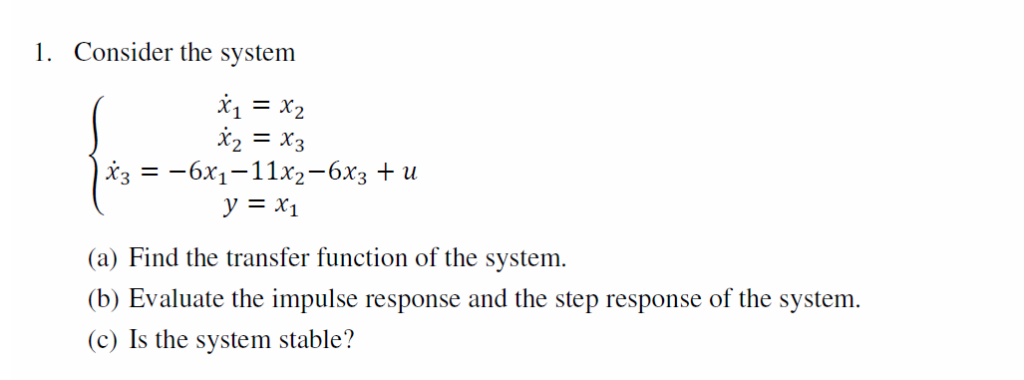1. Consider the system X2 X3 (a) Find the transfer function of the system. (b) Evaluate the impulse response and the step response of the system (c) Is the system stable?

• ### Question 1 (10 pts): Consider the continuous-time LTI system S whose unit impulse response h is given by Le., h consists of a unit impulse at time 0 followed by a unit impulse at time (a) (2pts)...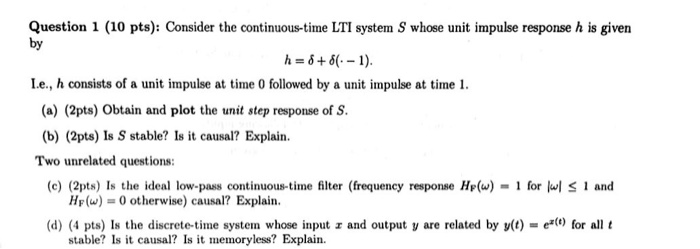Question 1 (10 pts): Consider the continuous-time LTI system S whose unit impulse response h is given by Le., h consists of a unit impulse at time 0 followed by a unit impulse at time (a) (2pts) Obtain and plot the unit step response of S. (b) (2pts) Is S stable? Is it causal? Explain Two unrelated questions (c) (2pts) Is the ideal low-pass continuous-time filter (frequency response H(w) for H()0 otherwise) causal? Explain (d) (4 pts) Is the discrete-time...

• ### a = 3 signals and systems 1) [10 pts. Let a system be defined as ta...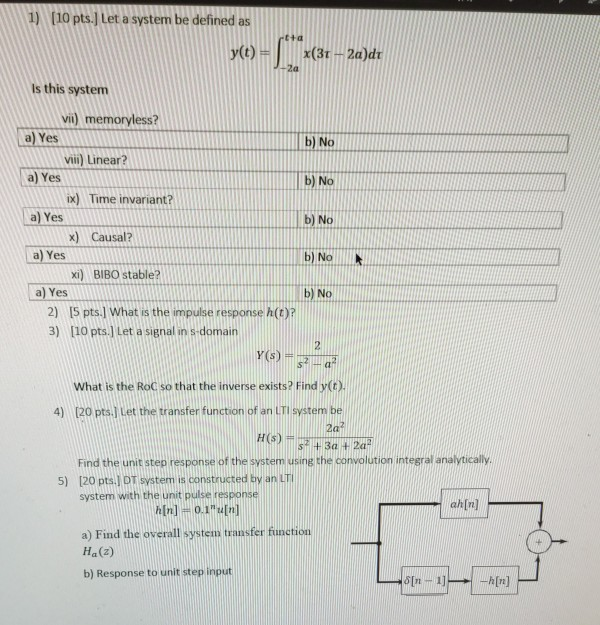a = 3 signals and systems 1) [10 pts. Let a system be defined as ta y(t) x(31 - 2a)dt 2a Is this system b) No b) No b) No vii) memoryless? a) Yes viii) Linear? a) Yes ix) Time invariant? a) Yes x) Causal? a) Yes xi) BIBO stable? a) Yes 2) [5 pts. What is the impulse response h(t)? 3) [10 pts.] Let a signal in s domain b) No b) No 2 Y(S) Sa What is the...

• ### Question 11 1 pts An LTI system is BIBO stable if and only if the impulse...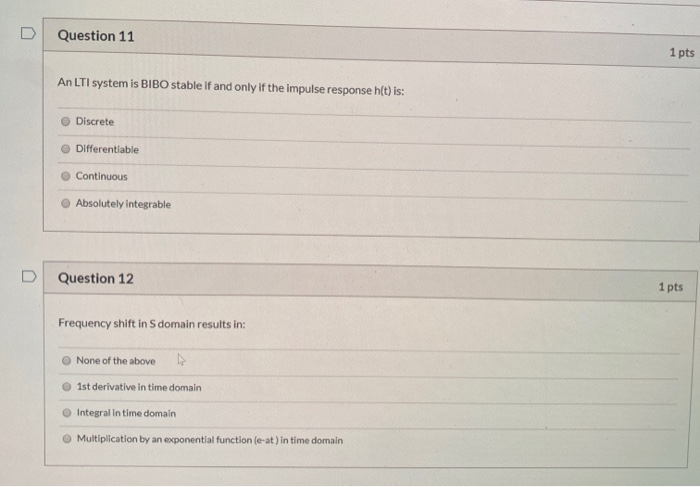Question 11 1 pts An LTI system is BIBO stable if and only if the impulse response h(t) is: Discrete Differentiable Continuous Absolutely integrable Question 12 1 pts Frequency shift in S domain results in: None of the above Ist derivative in time domain Integral in time domain Multiplication by an exponential function (e-at) in time domain

• ### 1. (4 pts) Consider the system whose transfer function is YS) – H(S) = Tos +1...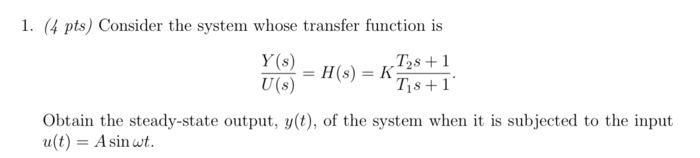1. (4 pts) Consider the system whose transfer function is YS) – H(S) = Tos +1 Tis +1 U(s) Obtain the steady-state output, y(t), of the system when it is subjected to the input u(t) = A sin wt.

• ### Find the zero-state response of the linear system with transfer function with an > 0 and 0 <くく1, when the input u(t) is the unit step, that is, u(t)-1(t), for 12 o, using both 1) the transfer...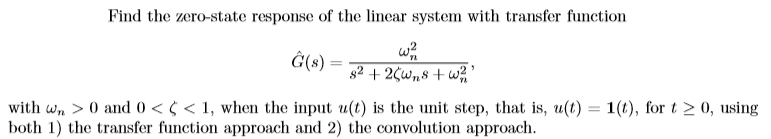Find the zero-state response of the linear system with transfer function with an > 0 and 0 <くく1, when the input u(t) is the unit step, that is, u(t)-1(t), for 12 o, using both 1) the transfer function approach and 2) the convolution approach Find the zero-state response of the linear system with transfer function with an > 0 and 0

• ### A unity feedback system with the forward transfer function G(s)=K/(s+1)(s+3)(s+6) is operating wi...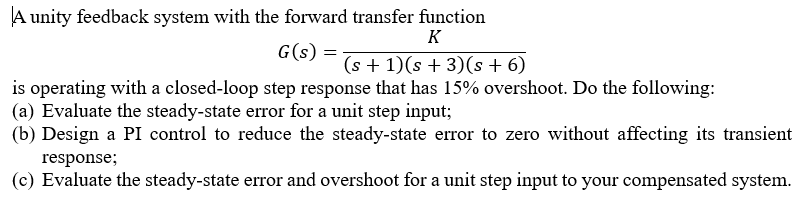A unity feedback system with the forward transfer function G(s)=K/(s+1)(s+3)(s+6) is operating with a closed-loop step response that has 15% overshoot. Do the following: a) Evaluate the steady-state error for a unit step input b) Design a PI control to reduce the steady-state error to zero without affecting its transient response c) Evaluate the steady-state error and overshoot for a unit step input to your compensated system A unity feedback system with the forward transfer function G(s) is operating with...

• ### Q4. 1 2 3 G 10 pts. Use MATLAB and plot the step response of the following systems G3 2s+1 figure. Gy on the same 2s+1...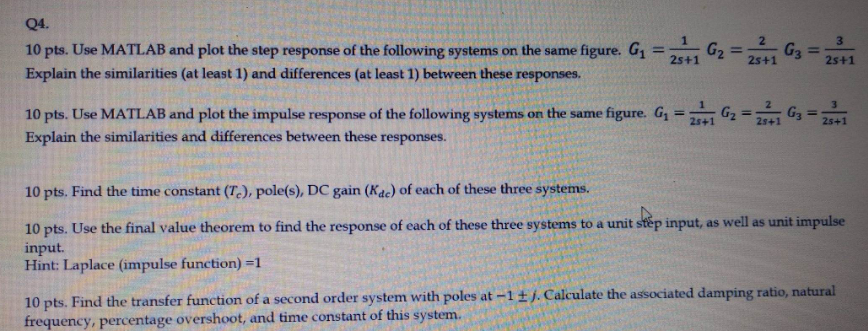Q4. 1 2 3 G 10 pts. Use MATLAB and plot the step response of the following systems G3 2s+1 figure. Gy on the same 2s+1 2s+1 Explain the similarities (at least 1) and differences (at least 1) between these responses. E_ figure. G, G 3 10 pts. Use MATLAB and plot the impulse response of the following systems Explain the similarities and differences between these responses. on the same 25+1 10 pts. Find the time constant (Te), pole(s), DC...

Free Homework App